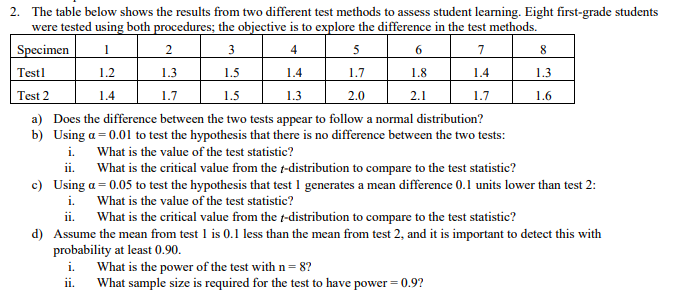# 2.The table below shows the results from two different test methods to assess student learning. Eight first-grade studentswere tested using both procedures; the objective is to explore the difference in the test methods6SpecimenTestlTest 21.22.01.6a)Does the difference between the two tests appear to follow a normal distribution?Using a 0.01 to test the hypothesis that there is no difference between the two tests:b)i.ii.What is the value of the test statistic?What is the critical value from the t-distribution to compare to the test statistic?c)Using u-0.05 to test the hypothesis that test I generates a mean difference 0.1 units lower than test 2i. What is the value of the test statistic?ii. What is the critical value from the t-distribution to compare to the test statistic?d) Assume the mean from test is 0.1 less than the mean from test 2, and it is important to detect this withprobability at least 0.90i. What is the power of the test with n-8?sample size is required for the test to havepower 0.9?

Question
1 viewshelp_outlineImage Transcriptionclose2. The table below shows the results from two different test methods to assess student learning. Eight first-grade students were tested using both procedures; the objective is to explore the difference in the test methods 6 Specimen Testl Test 2 1.2 2.0 1.6 a) Does the difference between the two tests appear to follow a normal distribution? Using a 0.01 to test the hypothesis that there is no difference between the two tests: b) i. ii. What is the value of the test statistic? What is the critical value from the t-distribution to compare to the test statistic? c) Using u-0.05 to test the hypothesis that test I generates a mean difference 0.1 units lower than test 2 i. What is the value of the test statistic? ii. What is the critical value from the t-distribution to compare to the test statistic? d) Assume the mean from test is 0.1 less than the mean from test 2, and it is important to detect this with probability at least 0.90 i. What is the power of the test with n-8? sample size is required for the test to have power 0.9? fullscreen
check_circle

Step 1

Hey, since there are multiple subparts posted, we will answer first three parts. If you want any specific part to be answered then please submit that question only or specify the subpart number in your message

a.

The population standard deviation of the difference between the two tests is unknown. Therefore, the difference between the two tests does not follow normal distribution. It will follow student’s t distribution with (n-1) degrees of freedom. n is the sample size.

Step 2

b.

The calculation for the test statistic:

Step 3

The standard deviation of the difference can be found by the EXCEL formula =STDEVA(Values). ...

### Want to see the full answer?

See Solution

#### Want to see this answer and more?

Solutions are written by subject experts who are available 24/7. Questions are typically answered within 1 hour.*

See Solution
*Response times may vary by subject and question.
Tagged in

### Hypothesis Testing